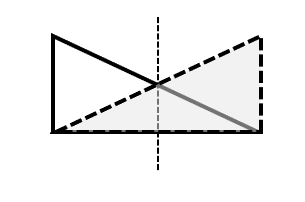Author:
Kimberly
Subject:
Secondary Mathematics
Material Type:
Homework/Assignment, Lesson
Level:
High School
Tags:
• Lesson Plan
• Polygons
• Reflection
• Reflectional Symmetry
• Rotation
• Rotational Symmetry
• Symmetry
• Transformation
Language:
English
Media Formats:
Text/HTML

# Rotational and Reflectional Symmetry of Polygons## Overview

This is a lesson plan that introduces rotational and reflectional symmetry with geometric shapes, focusing on regular polygons. Notes (two copies: a student version and also an answer key, both as a Word doc and a PDF), assignment, and cut-out shapes are provided.

# Summary

The following lesson is on rotational and reflectional symmetries of various regular polygons, including squares, rectangles, triangles, parallelograms, rhombi, hexagons, trapezoids and other shapes. Students will explore various reflections and rotations and determine if the image is congruent to the pre-image.

Materials

There are 2 pages of cut-out shapes to be used for a more visual representation of the rotations and reflections. These can be printed and cut out prior to class, or the students can cut them out themselves.

Brads may be used to allow for easier exploration of rotational symmetry if needed for demonstration purposes, or for students' individual exploration, especially for diverse learners.

String or popsicle sticks may be used to attach to the shapes for easier exploration of reflectional symmetry if needed for demonstration purposes, or for students' individual exploration, especially for diverse learners.

Students will need a straight edge to draw the line of reflection.

Calculators may be needed to calculate the angle of rotational symmetry.

Time Frame

1-2, 45 minute sessions.

# Background for Teachers

To teach this lesson, you will need an understanding of geometric transformations, specifically rotations and reflections. For example:

• Squares have both rotational (90o, 180o, 270o) and reflectional symmetries (vertical, horizontal, and diagonal).

How to Identify and Calculate Rotational Symmetry

Symmetry in Geometry

Here is an interactive webpage that rotates various shapes to set degrees (please note: the rotations here are counterclockwise, where the rotations in the notes and assignment are clockwise).

https://contrib.pbslearningmedia.org/WGBH/mgbh/mgbh-int-anglerota/index.html

There are also two copies of the notes and assignment. One is a student version, and the other is the teacher version which includes the answers.

# Step 1 - Goals and OutcomesLearning Intentions:

• Students will be able to understand rotational and reflectional translations of regular polygons.
• Students will be able to understand rotational and reflectional symmetries of regular polygons.

Success Criteria:

• Students will describe various rotational and reflectional symmetries of given regular polygons.
• Students will describe properties of the regular polygons based on these symmetries.

# Step 2 - Planning InstructionStudent Background Knowledge

Prior to this lesson, students will need to have an understading of

• Regular polygons and some basic properties
• Translations of lines and shapes
• Defintion of congruent geometric figures
• Linear equations
• Basic understanding of degrees and rotation

Strategies for Diverse Learners

Several pages are included in the lesson to assist in creating a more hands-on experience.

Different reflections can be drawn on the shapes with a dashed line. Or a popcicle stick or piece of string may be used as the line of reflection. Glue the popcicle stick or tape the string onto the back of the shape and use that to reflect the shape.

For rotations, connect two congruent images in the center with a brad. The students can then rotate the top image until it matches the bottome image to understand rotational symmetries.

Strategies and Process

Consider having the students work in groups of at least two, which will allow students to check their answers more easily. This is especially helpful for students who may not as easily identify symmetries (as opposed to similarities), as it is easy to think that some shapes have certain symmetries when they actually don't.

This would also work as a virtual lesson, as the student version of the notes includes the assignment and cutout shapes.

# Step 3 - Instruction# Step 4 - AssessmentsAssessment for this lesson will include an exit ticket.

Hold up a shape and ask the student if there is reflectional symmetry or rotational symmetry.

You could likewise hold up two images, one image and the other a pre-image and ask the student what type of transformation occurred and if there is any symmetry involved.